Reach Us+44 1704 335730
Free Vibration Analysis of Single-Walled Carbon Nanotubes Based on the Continuum Finite Element Method | OMICS International
Global Journal of Technology and Optimization
All submissions of the EM system will be redirected to Online Manuscript Submission System. Authors are requested to submit articles directly to Online Manuscript Submission System of respective journal.

# Free Vibration Analysis of Single-Walled Carbon Nanotubes Based on the Continuum Finite Element Method

Chandan Mungra and Jeffrey F Webb*

Department of Mechanical, Materials and Manufacturing Engineering, The University of Nottingham Malaysia Campus, Jalan Brog, 43500 Semenyih, Selangor, Malaysia

Corresponding Author:
Jeffrey F Webb
Department of Mechanical
Materials and Manufacturing Engineering
The University of Nottingham Malaysia Campus
Jalan Brog, 43500 Semenyih, Selangor, Malaysia
Tel: +60-3-8725-3482;
E-mail: [email protected]

Received date: January 06, 2015; Accepted date: January 06, 2015; Published date: January 16, 2015

Citation: Mungra C, Webb JF (2015) Free Vibration Analysis of Single-Walled Carbon Nanotubes Based on the Continuum Finite Element Method. Global J Technol Optim 6:173. doi: 10.4172/2229-8711.1000173

Copyright: © 2015 Mungra C, et al. This is an open-access article distributed under the terms of the Creative Commons Attribution License, which permits unrestricted use, distribution, and reproduction in any medium, provided the original author and source are credited.

Visit for more related articles at Global Journal of Technology and Optimization

#### Abstract

This paper presents a continuum finite element mechanics approach to model the vibration behaviours of single-walled carbon nanotubes (SWCNTs) of varying lengths, aspect ratios, chiralities, boundary conditions, axial loads and with initial strain applied. The results are in good agreement with the open literature and show that resonance-based carbon nanotubes sensors have the potential to meet the high level performance requirements inherent of many sensor based applications such as mass detectors, biomedical sensors, monitoring for metal deposition and chemical reactions amongst others. Currently, the sensitivity of many electromechanical transducers used for these applications have reached their respective theoretical limit. The merit of carbon nanotubes is that, due to their miniature dimensional structures, the sensitivity of these sensor based applications is vastly improved.

#### Keywords

Nanotubes, Free Vibration, Element Method, Single- Walled Carbon

#### Introduction

Carbon nanotubes (CNTs) are inherent of some of the most unique chemical and physical structures, conferring them very special mechanical, chemical, optical, thermal and electrical properties. CNTs therefore have a wide ranging application prospect [1-3]. This extends to newer pioneer structural materials in particular because amongst the excellent mechanical characteristics that CNTs possess include high elastic modulus, high toughness, high strength and a low density. In this way, they are very suitable and can be an effective component in NEMS/MEMS, as ultra-high frequency resonators and in composite materials [4,5]. Owing to their small sizes, single-walled carbon nanotubes (SWCNTs) have a large surface area to volume ratio and on external application of a mechanical deformation, they can respond quickly with a high sensitivity. Together with an ultra-high natural frequency inherent of SWCNTs, they can aid in the design and development of the fastest ever scanning probes, resonant magnetic force microscopes and even high-clocked supercomputers. While the mechanical properties results for SWCNTs and in particular the elastic modulus results predicted through research have shown wide acceptance, the predicted vibration behaviours are presented by varying models and theories and these can vary considerably. With regards to this, a simple yet reliable dynamic model is presented to analyze the vibration behavior of SWCNTs.

The use of experimental measurement methodology is a very costly approach and the results can often be very scattered because of the small size of SWCNTs. On the other hand, the analytical analysis approach is more cost-effective but is mostly applied to simple- structured CNTs showing simple mode deformations. In this way, numerical computer based approach is a powerful and efficient way for carrying out the analysis of the dynamic behaviours of CNTs in various configurations of parameters and applied boundary conditions .

Numerical models comprise of molecular dynamics and continuum mechanics models. Continuum mechanics analysis is amongst the simplest yet most efficient approach to study the static and dynamic behaviours of CNTs as compared to other such models so far. A space-frame structured model was proposed by Li and Chou  and implemented in this study to analyze dynamic vibration behaviours of SWCNTs with distinct lengths, chiralities, aspect ratios, boundary conditions, axial loads and with initial strain applied. The outcome shows that the results of this study are in agreement with the solutions obtained from the more sophisticated and complex molecular dynamics approach models.

#### Structure and Properties of Carbon Nanotubes

Two kinds of CNTs exist; these are single walled carbon nanotubes (SWCNTs) and multi-walled carbon nanotubes (MWCNTs). MWCNTs are SWCNTs with different radii that are coaxially interposed. The simplest way to imagine SWCNTs is by making reference to a rolled up grapheme sheet to produce a hollow cylinder but with end caps. Hexagonal carbon structures make up the cylinder, while pentagonal carbon structures make the end caps. Periodical repletion of the hexagonal patterns leads to the connection of every carbon atom to three other neighboring atoms. This connection is called a covalent bond and it confers the structure its impressive mechanical characteristics by virtue of being a strong chemical bond.

Chirality, which is characterized by a chiral vector Cn and a chiral angle θ, determines which atomic structure the CNT takes. The chiral vector is the line joining crystallographically equivalent parallel regions of O and C atom in a flat 2D grapheme sheet (Figure 1). Lattice translation indices (n,m) together with the vectors α1 and α2 in the hexagonal lattice, defines the Ch vector with the equivalent equation being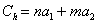The angle this chiral vector makes relative to a zigzag direction (n,0) defines the chiral angle θ. Armchair nanotubes are formed when the chiral angle is equivalent to 0° whilst zigzag nanotubes occur at a chiral angle of 30°. They both are limiting cases with (n,n) characterising armchair nanotubes while (n,0) corresponds to zigzag ones. When the chiral angles are not 0°or 30°, chiral nanotubes are formed and they bear the indices (n,m) where n≠m (Figure 2).

#### Structural Continuum Mechanics Model

In the carbon nanotubes geometrical structure, neighboring carbon atoms are interconnected by covalent bonds such that they form the hexagonal lattice structure at the walls. In a 3D space, these have a certain characteristic bond length as well as bond angles (Figure 3). On application of an external force, the covalent bonds constraint the motion of individual atoms of CNT. Thus the net deformation is due to the interactions of these covalent bonds. By applying an analogy between these bonds and connecting elements occurring between neighboring carbon atoms, a CNT can be regarded as a finite element space frame structure. The individual atoms would then act as joints between connected elements. The correlation linking the parameters of sectional stiffness of structural mechanics to the force constants of molecular mechanics is then established. The bond sections are assumed to be uniformly circular in shape and identical . This leads to lx = ly= l with only the stiffness parameter values ‘EA’, ‘EI’ and ‘GJ’ are required to be solved for.

Since deformation of the space frame model causes a change in the strain energies, the stiffness parameter values can be determined through energy equivalence. Then, as per structural mechanics [6,7] theory, for a uniform beam of length L with an axial force N acting on it, the strain energy I given by: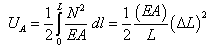(1)

where ΔL represents deformation due to axial stretching.

For a uniform beam under bending moment M (Figure 4), the strain energy is given by: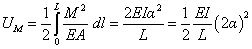(2)

Where a represents the rotational angle at the beam endings.

For a uniform beam under torsion T,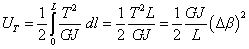(3)

where Δβ represents the relative rotations at the beam endings.

Also merging dihedral angular torsions and improper torsions in a singular term, the simple harmonic forms are given as follows: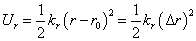(4)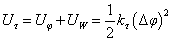(5)(6)

where kr is the force constant for bond stretching, kθ is the force constant for bending of the bond angles and kτ is the torsional resistance. Δr, Δθ and Δφ are the increment in bond stretching, change in bond stretching, change in bond angle and the change in angle for the case of bond twisting, respectively.

From equations (1) to (6), the stretching energies are given by Ur and UA . Uθ and UM are the bending energies and U_τ and UT are the torsional energy. It could then be assumed that the angle of rotation 2α is equal to the net change Δθ of the bond angles, ΔL is equal to Δr and Δβ is equal to Δφ. Comparing the equations, the structural mechanics values ‘EA’, ‘El’ and ‘GJ’ and the molecular mechanics parameters kr, kθ, kτ and kτ, it can be found that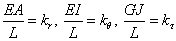(7)

Equation (7) illustrates the application of structural mechanics to modeling for the CNT. Therefore, if values of kr, kθ, and kτ are known, the stiffness parameters ‘EA’, ‘EI’ and ‘GJ’ can be found easily. Then by making use of the procedure to find the stiffness matrix for framelike structures, determination of static and dynamic responses can be appropriately simulated.

As mentioned earlier, carbon nanotubes consist of atoms interconnected by covalent bonds to form a hexagonal lattice structure. They can be characterised by a certain bond length ac-c and a bond angle in a three-dimensional space. Therefore the bonds constrain the displacement of these individual atoms while they are subjected to vibration dynamics. In this way, the bonds can be considered as interconnected load-carrying elements while the atoms as joints. This in effect makes CNTs analogous to space-frame structures.

Considering the SWCNTs as space-frame structured equivalent models to solve for natural frequencies under free undamped vibration, the equation of motion takes the following form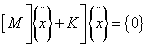(8)

where [M] is the global mass matrix, [K] is the stiffness matrix representing nodal displacement and acceleration vectors respectively. Neglecting the electron masses, the carbon nuclei mass (mc= 1.993×10-26 kg) is assumed to be effectively concentrated at the atomic centers. In this way the inertial atomistic property of CNTs is taken into account.

By using this methodology in the project, basic structural mechanics could determine the mechanical behaviour of CNTs. Using the ANSYS finite element module, three-dimensional finite element models of CNTs are created for analysis in this project. Beam188 3D elastic ANSYS elements are used to mimic the bonds. These are uniaxial and allow for compression, bending as well as torsional characteristics. Each of them have six degrees of freedom at the node allowing for x, y, z directional translations as well as rotation along these axes. These elements can be described as having a certain cross-sectional area, two moments of inertia, the inherent material properties, and constrained by 2 to 3 nodes.

The hexagonal nanostructure present in CNTs (Figure 5) can thus be simulated as a space-frame structure. An extension to this approach allows for the simulation of the entire CNT lattice structure. The length of the element L corresponds to the bond length, αc-c and the thickness of the element represents the wall thickness t, which by assuming the cross-sectional profile of the element to be circular, is analogous to the element diameter d.

While CNTs occur as hollow tubes with closed end- caps at their extremities, open ended CNTs would be modelled so as to simplify the analysis. Using ANSYS macro language a cyclic procedure was established for generation. This is carried out by inputting individual Cartesian coordinates of atoms of carbon through using JCRYSTAL© software to determine exact values and inputting the value of the bond length at the equilibrium state.

Following this methodology, the carbon atom coordinates generate the nodes in the finite element model and eventually the beam elements between them. These equivalent space-frame structures were then solved to find their fundamental frequencies. Figure 6 depicts the side lateral views of the finite element meshes armchair, zigzag and chiral single- walled carbon nanotubes.

#### Structural Continuum Mechanics Model

Accurate theoretical analysis models are very important when determining the fundamental frequencies and associated mode shapes for several reasons. When it comes to nanostructure resonators for example, the fundamental oscillation frequencies are key attributes of the associated resonator. In addition to that, the natural frequency and mode shape results, if an adequately accurate theoretical analysis model is used, allows the determination of key mechanical characteristics of CNTs such as the elastic modulus. In this section, the effects of varying length, aspect ratio, chirality, boundary conditions, axial loads and initial strain on the fundamental frequencies of SWCNTs have been studied and compared quantitatively with molecular dynamics results as well to vouch the accuracy. All frequency results presented in this study are in THz unless otherwise stated.

Length

Length is one of the key attributes that affect the natural frequencies of a SWCNT. An armchair type (10,10) and zigzag (17, 0) SWCNT with vary aspect ratios from 8.28 to 39.1 were analyzed. These chiralities have been applied so that their diameters would be approximately the same. The first vibration mode frequencies were extracted for each of them and the results were tabulated and compared with the literature values . Overall it can be seen that an increase in the length causes the natural frequencies for the first mode to decrease which is agreeable to the open literature. Figures 7 and 8 illustrate how the equivalent spaceframe beam model performs against molecular dynamics results. It can be seen that the values are nearer to the MD results when the length-todiameter ratio is greater than 10.07. Also the difference in chirality does not affect the fundamental frequencies considerably. The relatively slightly higher values for the armchair (10, 10) SWCNT compared to the zigzag (17, 0) one may be accounted by virtue of the chiralitydependent anisotropic nature of the carbon nanotubes structure.

Aspect ratio

As depicted in Figures 7 and 8, an increase in the length to diameter ratio causes a decrease in the fundamental frequencies of the SWCNTs. But what is also prevalent, is that the aspect ratio is a key attribute in determining whether the SWCNT takes a bending or breathing mode shape. Figure 9 illustrates the first five resulting mode shapes for a SWCNT of length 70 Å and chirality of (7, 7). The first and second mode shapes take both a half-sine wave shape while the last three take the shape of a breathing wave.

Increasing the aspect ratio by simulating a (7, 7) SWCNT of length 50Å shows different results with the higher modes bearing breathing wave shaped modes. These simulations show a trend whereby increasing the SWCNT length causes breathing wave mode shapes to be weakened and the bending wave mode shapes to become more dominant as shown in Figure 10.

Chirality

Further tests have been performed to determine the effect of varying the chirality of SWCNTs on the resulting fundamental frequencies. The chiralities applied in Table 1 have been chosen so that the SWCNTs have roughly the same diameter. It can be seen from Tables 1 and 2 that the natural frequencies obtained are approximately the same, that is, the results are independent of the chiral angle magnitudes to a considerable degree. This agrees with the observations by Li and Chou  who used the molecular dynamics approach.

(n,m) Chiral Angle MD (THz) Results (THz)
(9, 0) 0.00 0.05493 0.048278 −12.11
(8, 2) 10.89 0.05493 0.047899 −12.80
(7, 3) 17.00 0.05493 0.048042 −12.54
(6, 4) 23.41 0.05493 0.048349 −11.98
(5, 5) 30.00 0.05493 0.047954 −12.70

Table 1: Results and comparison of the chiralities for the 1st mode.

(n,m) Chiral Angle MD (THz) Results (THz)
(9, 0) 0.00 0.76904 0.701826 −8.74
(8, 2) 10.89 0.75684 0.691373 −8.65
(7, 3) 17.00 0.76904 0.703595 −8.51
(6, 4) 23.41 0.78735 0.719795 −8.58
(5, 5) 30.00 0.80556 0.734590 −8.81

Table 2: Results and comparison of the chiralities for the 3rd mode.

Next, the mode shapes of SWCNTs with different chiralities were investigated. Figure 11 illustrates the first three mode shapes of chiral and zigzag SWCNTs. It can be seen that the chiral and zigzag modeshapes are interchanged from breathing to bending largely due to a change in length, further showing the insensitivity of chirality but greater sensitivity to the length-to-diameter ratio. These CNT mode shapes are very important criteria to be considered when designing CNT associated devices.

Figure 11: The first three vibration modes of SWCNTs having different chirality: (a) (11,0) SWCNT in a length of 45Å; (b) (11,0) SWCNT in a length of 55Å; (c) (8,4) SWCNT in a length of 40Å; (d) (8,4) SWCNT in a length of 50Å.

Boundary conditions

The natural frequencies of armchair SWCNTs with a chirality of (5,5) with a clamped-free (CF) boundary conditions and clampedclamped (CC) conditions were investigated. Figure 12 illustrates these sets of conditions. The results for the clamped-free conditions show that there is a smooth decrease in the natural frequencies as the aspect ratios increase incrementally for each mode and that the higher the modes, the higher are the resulting frequencies as shown in Figure 13. Since these SWCNTs have almost identical diameters, the inverse proportionality between the fundamental frequencies and aspect ratio implies that a longer SWCNT tends to show decreased sensitivity to the resulting frequencies. The results are in good agreement with the MD values in the open literature showing the same trend, with the fundamental frequencies nearer to MD results as from an aspect ratio of 7.55 onwards. Clamped grapheme sheets and CNTs are often the configuration of choice for studying the mechanical properties of carbon nanotubes both with atomistic approach and experimental studies.

One the other hand, clamped-clamped SWCNTs configurations are principally applied to nanostrain sensors as well as micro-oscillators. For comparison reasons, the same (5, 5) armchair SWCNT for CF conditions was applied to the clamped-clamped condition. Figures 14 and 15 shows the obtained frequency results and comparison with molecular dynamics results. As with CF condition, the SWCNTs tend to show inverse proportionality between the natural frequencies and corresponding aspect ratio. However the introduction of the new boundary condition shows much higher frequency results compared to the CF condition which is definitely an asset for CNT-based micro-oscillators.

Initial strain

The natural frequencies of CNTs display high sensitivity to externally applied loads. Resonant strain sensors based on CNTs make use of this principle. It is therefore important to find the relationship between resonant frequencies of CNTs and stress conditions applied. The vibration behavior when a compressive or tensile initial strain is present is essential to fulfill CNTs’ prospect as nanosensors. The simulations are carried out on a SWCNT having a chirality of (5, 5) and aspect ratio of 13.89, with tensile or compressive strains applied to determine its response towards resonant frequencies. The obtained results (Figures 16 and 17) show the same trend as the MD results. Figure 18 shows that initial tensile strains tend to cause an increase in the frequencies while initial compressive strains tend to cause a decrease of the frequencies. This agrees with continuum vibration theory predictions .

#### Conclusion

In this paper, the free vibration analysis of SWCNTs based on the continuum finite element model, with varying lengths, aspect ratios, chiralities, boundary conditions, axial loads and initial strain condition were performed. It was found that an increase in the length or aspect ratio would cause a smooth decrease in the fundamental frequencies and that the higher frequencies occurred at higher modes of vibration. It was also observed that the aspect ratio is a key element in determining whether the vibration mode took a bending or breathing wave shape. The results have shown that increasing the aspect ratio would cause breathing mode shapes to weaken while bending or half-sine wave mode shapes to become more prominent. Since these mode shapes are key criteria when designing CNT associated devices, it highlights the reason as to why in nanotubes synthesis, uniformity control is so important, especially for CNT based vibration sensors and resonators. The results for chirality showed that a change in the chiral angle magnitude would not cause any considerable change in the results and it is agreeable to the open literature. For a clamped-free SWCNT, an inverse proportionality relationship was observed between the resulting fundamental frequency and aspect ratio. The results took a similar trend for clamped-clamped SWCNTs but much higher frequency values were obtained in this case and this is definitely an asset for CNT-based micro- oscillators. Moreover the application of an axial load in the midsection of SWCNTs showed that the logarithmic relationship between frequencies and aspect ratio could be used as mass sensing devices for quantifying nano-particle size. Shorter size CNTs tend to have increased sensitivity. The introduction of initial strains show that initial compressive strains lead to a reduction in frequencies while initial tensile strains resulted in an increase in frequencies, which agrees well with continuum vibration theory. Overall, most of the results are in good agreement with the more complex and sophisticated molecular dynamics literature results and in terms of relative simplicity and efficiency the equivalent space-frame finite element model used performed aptly.

#### References

Select your language of interest to view the total content in your interested language

### Article Usage

• Total views: 13166
• [From(publication date):
June-2015 - Nov 14, 2019]
• Breakdown by view type
• HTML page views : 9190Can't read the image? click here to refresh